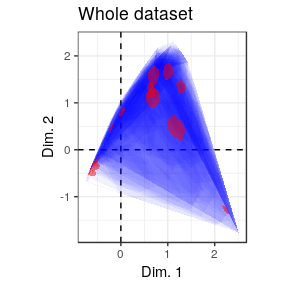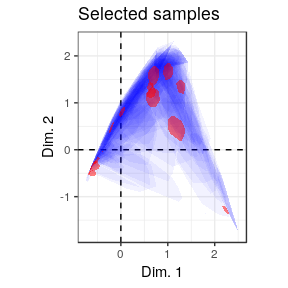# Matrix seriation

## Introduction

The matrix seriation problem in archaeology is based on three conditions and two assumptions, which Dunnell (1970) summarizes as follows.

The homogeneity conditions state that all the groups included in a seriation must:

• Be of comparable duration,
• Belong to the same cultural tradition,
• Come from the same local area.

The mathematical assumptions state that the distribution of any historical or temporal class:

• Is continuous through time,
• Exhibits the form of a unimodal curve.

Theses assumptions create a distributional model and ordering is accomplished by arranging the matrix so that the class distributions approximate the required pattern. The resulting order is infered to be chronological.

## Seriation

The following methods assume that you keep your data tidy: each variable (taxon/type) must be saved in its own column and each observation (sample/case) must be saved in its own row. Missing values are not allowed.

### Reciprocal ranking

Reciprocal ranking iteratively rearrange rows and/or columns according to their weighted rank in the data matrix until convergence (Ihm 2005).

We denote the $$m \times p$$ incidence matrix by $$C = \left[ c_{ij} \right] ~\forall i \in \left[ 1,m \right], j \in \left[ 1,p \right]$$ with row and column sums:

\begin{align} c_{i \cdot} = \sum_{j = 1}^{p} c_{ij} && c_{\cdot j} = \sum_{i = 1}^{m} c_{ij} && c_{\cdot \cdot} = \sum_{i = 1}^{m} \sum_{j = 1}^{p} c_{ij} && \forall c_{ij} \in \lbrace 0,1 \rbrace \end{align}

The rows of $$C$$ are rearranged in increasing order of:

$x_{i} = \sum_{j = 1}^{p} j \frac{c_{ij}}{c_{i \cdot}}$

The columns of $$C$$ are rearranged in a similar way:

$y_{j} = \sum_{i = 1}^{m} i \frac{c_{ij}}{c_{\cdot j}}$ These two steps are repeated until convergence.

Note that this procedure could enter into an infinite loop. If no convergence is reached before the maximum number of iterations, it stops with a warning.

# Build an incidence matrix with random data
incidence1 <- IncidenceMatrix(data = sample(0:1, 400, TRUE, c(0.6, 0.4)),
nrow = 20)

# Get seriation order on rows and columns
# Correspondance analysis-based seriation
(indices <- seriate(incidence1, method = "correspondance", margin = c(1, 2),
stop = 100))
#> Permutation order for matrix seriation:
#>    Row order: 14 10 19 4 6 20 13 3 1 9 5 17 8 16 18 11 7 15 2 12
#>    Column order: 18 7 1 10 19 14 13 5 8 9 20 3 15 16 4 12 6 11 17 2
#>    Method: correspondance

# Permute matrix rows and columns
incidence2 <- permute(incidence1, indices)

# Plot matrix
plotMatrix(incidence1) +
labs(title = "Original matrix") +
theme(legend.position = "bottom") +
scale_fill_manual(values = c("TRUE" = "black", "FALSE" = "white"))
plotMatrix(incidence2) +
labs(title = "Rearranged matrix") +
theme(legend.position = "bottom") +
scale_fill_manual(values = c("TRUE" = "black", "FALSE" = "white"))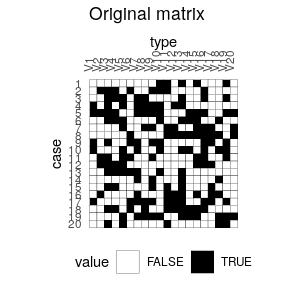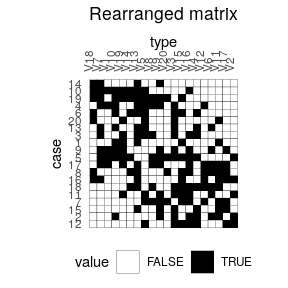### Reciprocal averaging

# Reproduces Desachy 2004 results
## Coerce dataset to an abundance matrix
comp1 <- as(compiegne, "CountMatrix")

## Plot original data matrix
plotBar(comp1, EPPM = TRUE) +
labs(title = "Original dataset") + theme_bw() +
theme(panel.spacing = unit(0, "lines"),
panel.border = element_rect(colour = NA),
legend.position = "bottom")

## Get seriation order for columns on EPPM using the reciprocal averaging method
## Expected column order: N, A, C, K, P, L, B, E, I, M, D, G, O, J, F, H
indices <- seriate(comp1, method = "reciprocal", EPPM = TRUE, margin = 2)

## Permute columns
comp2 <- permute(comp1, indices)

## Plot new matrix
plotBar(comp2, EPPM = TRUE) +
labs(title = "Reordered dataset") + theme_bw() +
theme(panel.spacing = unit(0, "lines"),
panel.border = element_rect(colour = NA),
legend.position = "bottom")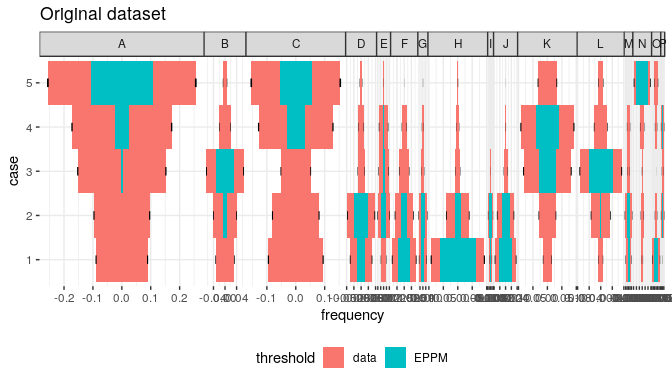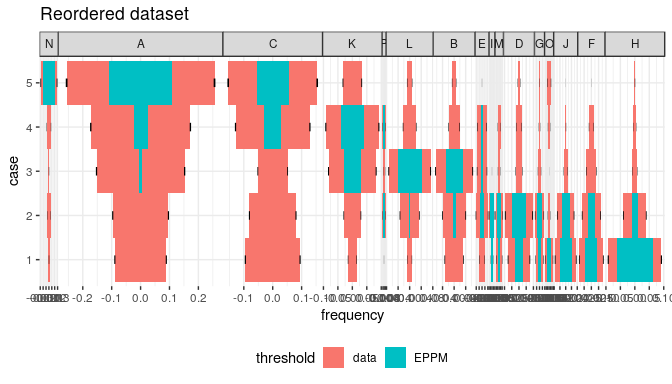### Correspondance analysis

Correspondance Analysis (CA) is an effective method for the seriation of archaeological assemblages. The order of the rows and columns is given by the coordinates along one dimension of the CA space, assumed to account for temporal variation. The direction of temporal change within the correspondance analysis space is arbitrary: additional information is needed to determine the actual order in time.

## Coerce dataset to an abundance matrix
zuni1 <- as(zuni, "CountMatrix")

# Correspondance analysis of the whole dataset
ca <- FactoMineR::CA(zuni1, graph = FALSE)

# Plot CA results
ggplot(mapping = aes(x = Dim 1, y = Dim 2)) +
geom_vline(xintercept = 0, linetype = 2) +
geom_hline(yintercept = 0, linetype = 2) +
geom_point(data = as.data.frame(ca$row$coord), color = "black") +
geom_point(data = as.data.frame(ca$col$coord), color = "red") +
coord_fixed() + theme_bw()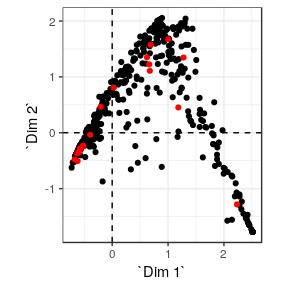# Get row permutations from CA coordinates
indices <- seriate(zuni1, method = "correspondance", margin = 1)

# Permute data matrix
zuni2 <- permute(zuni1, order = indices)

# Plot Ford diagram
# Warning: this may take a few seconds!
plotBar(zuni2, level = FALSE) +
theme(axis.text = element_blank(),
axis.ticks = element_blank(),
axis.title = element_blank())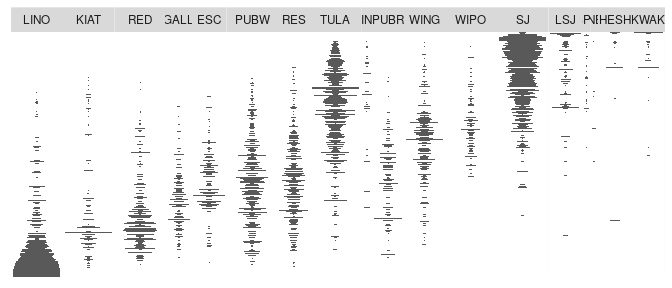#### Refine CA-based seriation

Peeples and Schachner (2012) propose a procedure to identify samples that are subject to sampling error or samples that have underlying structural relationships and might be influencing the ordering along the CA space. This relies on a partial bootstrap approach to CA-based seriation where each sample is replicated n times. The maximum dimension length of the convex hull around the sample point cloud allows to remove samples for a given cutoff value.

# Reproduces Peeples and Schachner 2012 results

## Samples with convex hull maximum dimension length greater than the cutoff
## value will be marked for removal.
## Define cutoff as one standard deviation above the mean
fun <- function(x) { mean(x) + sd(x) }

## Get indices of samples to be kept
## Warning: this may take a few seconds!
keep <- refine(zuni1, cutoff = fun, n = 1000)

## Plot convex hull
## blue: convex hull for samples; red: convex hull for types
### All bootstrap samples
ggplot(mapping = aes(x = x, y = y, group = id)) +
geom_vline(xintercept = 0, linetype = 2) +
geom_hline(yintercept = 0, linetype = 2) +
geom_polygon(data = keep[["rows"]], fill = "blue", alpha = 0.05) +
geom_polygon(data = keep[["columns"]], fill = "red", alpha = 0.5) +
coord_fixed() + theme_bw() + labs(title = "Whole dataset",
x = "Dim. 1", y = "Dim. 2")
### Only retained samples
ggplot(mapping = aes(x = x, y = y, group = id)) +
geom_vline(xintercept = 0, linetype = 2) +
geom_hline(yintercept = 0, linetype = 2) +
geom_polygon(data = subset(keep[["rows"]], id %in% names(keep[["keep"]])),
fill = "blue", alpha = 0.05) +
geom_polygon(data = keep[["columns"]], fill = "red", alpha = 0.5) +
coord_fixed() + theme_bw() + labs(title = "Selected samples",
x = "Dim. 1", y = "Dim. 2")